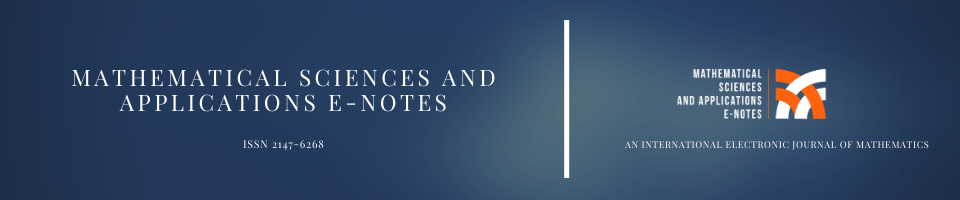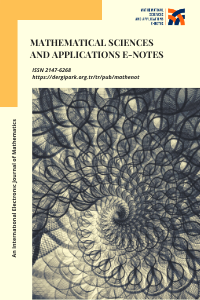##### Mathematical Sciences and Applications E-NotesMakale Gönder Hakemlik İsteği Gönder Amaç ve Kapsam Yazım Kuralları Etik İlkeler ve Yayın Politikası Son Sayı Editör Kurulu İletişim Arşiv
e-ISSN 2147-6268 | Yayın Aralığı Yılda 4 Sayı | Başlangıç: 2013 | Yayıncı Murat TOSUN | Math. Sci. Appl. E-NotesFrom 2021 onwards, Mathematical Sciences and Applications E-Notes (MSAEN), will be published four times a year, at intervals of three months, and only in online version.Refreshing the Aim and Scope of the Journal...

The editors have reviewed and adjusted the aims and scope of Mathematical Sciences and Applications E-Notes (MSAEN). The journal’s central mission is to publish research of outstanding significance and timeliness. We are now providing more detailed guidance on the most important topics and areas that will be emphasized in the future in order to achieve this goal.

The Journal welcomes papers on:

• Approximation theory, iteration methods and their applications
• Applied functional analysis and operator theory
• Real and harmonic analysis
• Complex analysis
• Numerical analysis
• Interpolation theory
• Optimization theory
• Functional, ordinary and partial differential equations
• Linear and nonlinear integral equations
• Numerical solution of differential and integral equations
• Fourier approximation
• Numerical linear algebra
• Applied mathematics

## Mathematical Sciences and Applications E-Notes

e-ISSN 2147-6268 | Yayın Aralığı Yılda 4 Sayı | Başlangıç: 2013 | Yayıncı Murat TOSUN | Math. Sci. Appl. E-NotesFrom 2021 onwards, Mathematical Sciences and Applications E-Notes (MSAEN), will be published four times a year, at intervals of three months, and only in online version.Refreshing the Aim and Scope of the Journal...

The editors have reviewed and adjusted the aims and scope of Mathematical Sciences and Applications E-Notes (MSAEN). The journal’s central mission is to publish research of outstanding significance and timeliness. We are now providing more detailed guidance on the most important topics and areas that will be emphasized in the future in order to achieve this goal.

The Journal welcomes papers on:

• Approximation theory, iteration methods and their applications
• Applied functional analysis and operator theory
• Real and harmonic analysis
• Complex analysis
• Numerical analysis
• Interpolation theory
• Optimization theory
• Functional, ordinary and partial differential equations
• Linear and nonlinear integral equations
• Numerical solution of differential and integral equations
• Fourier approximation
• Numerical linear algebra
• Applied mathematics